Test: Electromagnetic Wave Propagation

# Test: Electromagnetic Wave Propagation

Test Description

## 20 Questions MCQ Test GATE ECE (Electronics) 2023 Mock Test Series | Test: Electromagnetic Wave Propagation

Test: Electromagnetic Wave Propagation for Railways 2023 is part of GATE ECE (Electronics) 2023 Mock Test Series preparation. The Test: Electromagnetic Wave Propagation questions and answers have been prepared according to the Railways exam syllabus.The Test: Electromagnetic Wave Propagation MCQs are made for Railways 2023 Exam. Find important definitions, questions, notes, meanings, examples, exercises, MCQs and online tests for Test: Electromagnetic Wave Propagation below.
Solutions of Test: Electromagnetic Wave Propagation questions in English are available as part of our GATE ECE (Electronics) 2023 Mock Test Series for Railways & Test: Electromagnetic Wave Propagation solutions in Hindi for GATE ECE (Electronics) 2023 Mock Test Series course. Download more important topics, notes, lectures and mock test series for Railways Exam by signing up for free. Attempt Test: Electromagnetic Wave Propagation | 20 questions in 60 minutes | Mock test for Railways preparation | Free important questions MCQ to study GATE ECE (Electronics) 2023 Mock Test Series for Railways Exam | Download free PDF with solutions
 1 Crore+ students have signed up on EduRev. Have you?
Test: Electromagnetic Wave Propagation - Question 1

### At 50 MHz a lossy dielectric material is characterized by μ = 2.1μo ,ε = 3.6 εo and σ = 0.08 S/m. The electric field is Es = 6e-jγx uz V/m Que: The propagation constant γ is

Detailed Solution for Test: Electromagnetic Wave Propagation - Question 1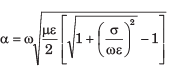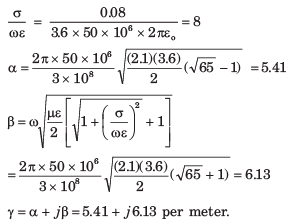Test: Electromagnetic Wave Propagation - Question 2

### At 50 MHz a lossy dielectric material is characterized by μ = 2.1μo ,ε = 3.6 εo and σ = 0.08 S/m. The electric field is Es = 6e-jγx uz V/m Que: The impedance η is

Detailed Solution for Test: Electromagnetic Wave Propagation - Question 2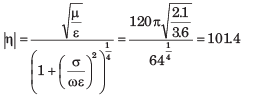Test: Electromagnetic Wave Propagation - Question 3

### A non magnetic medium has an intrinsic impedance 360∠30o Ω Que: The loss tangent is

Detailed Solution for Test: Electromagnetic Wave Propagation - Question 3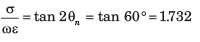Test: Electromagnetic Wave Propagation - Question 4

A non magnetic medium has an intrinsic impedance 360∠30o Ω

Que: The Dielectric constant is

Detailed Solution for Test: Electromagnetic Wave Propagation - Question 4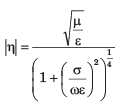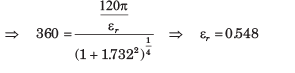Test: Electromagnetic Wave Propagation - Question 5

The amplitude of a wave traveling through a lossy nonmagnetic medium reduces by 18% every meter. The wave operates at 10 MHz and the electric field leads the magnetic field by 24o

Que: The propagation constant is

Detailed Solution for Test: Electromagnetic Wave Propagation - Question 5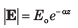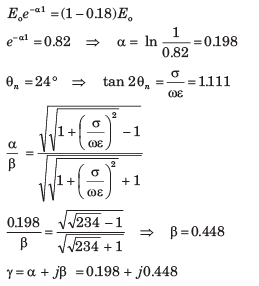Test: Electromagnetic Wave Propagation - Question 6

The amplitude of a wave traveling through a lossy nonmagnetic medium reduces by 18% every meter. The wave operates at 10 MHz and the electric field leads the magnetic field by 24o

Que: The skin depth is

Detailed Solution for Test: Electromagnetic Wave Propagation - Question 6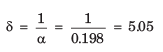Test: Electromagnetic Wave Propagation - Question 7

A 60 m long aluminium (σ = 3.5 x 107 S/m, μr = 1, ε2 = 1) pipe with inner and outer radii 9 mm and 12 mm carries a total current of 16 sin(106 πt)A. The effective resistance of the pipe is

Detailed Solution for Test: Electromagnetic Wave Propagation - Question 7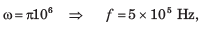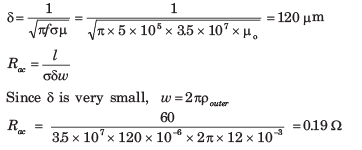Test: Electromagnetic Wave Propagation - Question 8

Silver plated brass wave guide is operating at 12 GHz. If at least the thickness of silver ( σ = 6.1 x 107 S/m, μr = εr = 1 ) is 5 δ the minimum thickness required for wave-guide is

Detailed Solution for Test: Electromagnetic Wave Propagation - Question 8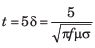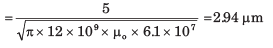Test: Electromagnetic Wave Propagation - Question 9

A uniform plane wave in a lossy nonmagnetic media has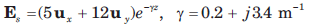The magnitude of the wave at z = 4 m and t = T/8 is

Detailed Solution for Test: Electromagnetic Wave Propagation - Question 9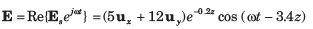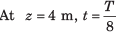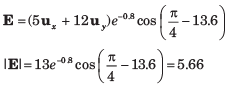Test: Electromagnetic Wave Propagation - Question 10

A uniform plane wave in a lossy nonmagnetic media hasQue: The loss suffered by the wave in the interval 0 < z < 3 m is

Detailed Solution for Test: Electromagnetic Wave Propagation - Question 10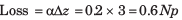1 Np =  8.686 DB, 0.6 Np = 5.21 dB.

Test: Electromagnetic Wave Propagation - Question 11

Region 1, z < 0 and region 2, z > 0, are both perfect dielectrics. A uniform plane wave traveling in the uz direction has a frequency of 3 x 1010 rad /s. Its wavelength in the two region are  λ1 = 5 cm and  λ2 = 3 cm.

Que: On the boundary the reflected energy is

Detailed Solution for Test: Electromagnetic Wave Propagation - Question 11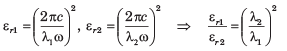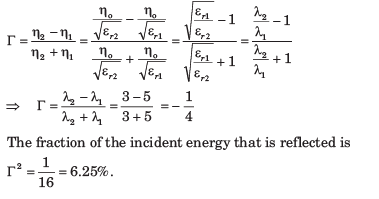Test: Electromagnetic Wave Propagation - Question 12

Region 1, z < 0 and region 2, z > 0, are both perfect dielectrics. A uniform plane wave traveling in the uz direction has a frequency of 3 x 1010 rad /s. Its wavelength in the two region are  λ1 = 5 cm and  λ2 = 3 cm.

Que: The SWR is

Detailed Solution for Test: Electromagnetic Wave Propagation - Question 12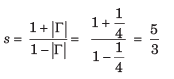Test: Electromagnetic Wave Propagation - Question 13

A uniform plane wave is incident from region 1 ( μr = 1, σ = 0) to free space. If the amplitude of incident wave is one-half that of reflected wave in region, then the value of εr is

Detailed Solution for Test: Electromagnetic Wave Propagation - Question 13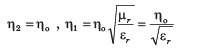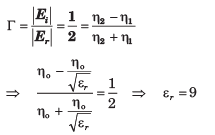Test: Electromagnetic Wave Propagation - Question 14

A 150 MHz uniform plane wave is normally incident from air onto a material. Measurements yield a SWR of 3 and the appearance of an electric field minimum at 0.3λ in front of the interface. The impedance of material is

Detailed Solution for Test: Electromagnetic Wave Propagation - Question 14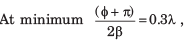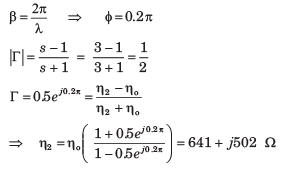Test: Electromagnetic Wave Propagation - Question 15

A plane wave is normally incident from air onto a semi-infinite slab of perfect dielectric (εr = 3.45) . The fraction of transmitted power is

Detailed Solution for Test: Electromagnetic Wave Propagation - Question 15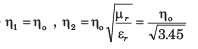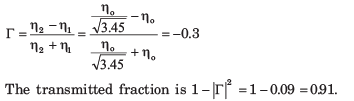Test: Electromagnetic Wave Propagation - Question 16

Consider three lossless region :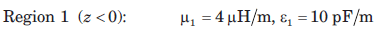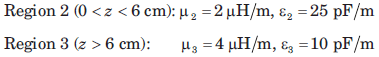Que: The lowest frequency, at which a uniform plane wave incident from region 1 onto the boundary at z = 0 will have no reflection, is

Detailed Solution for Test: Electromagnetic Wave Propagation - Question 16

This frequency gives the condition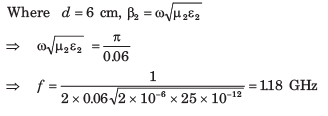Test: Electromagnetic Wave Propagation - Question 17

Consider three lossless region :Que: If frequency is 50 MHz, the SWR in region 1 is

Detailed Solution for Test: Electromagnetic Wave Propagation - Question 17

At 50 MHz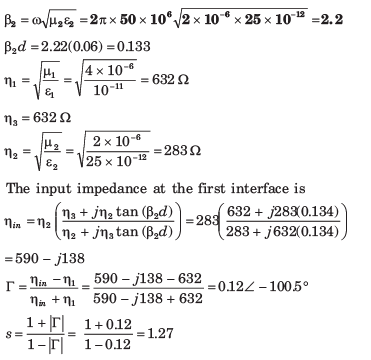Test: Electromagnetic Wave Propagation - Question 18

A uniform plane wave in air is normally incident onto a lossless dielectric plate of thickness  λ/8 , and of intrinsic impedance  η = 260 Ω. The SWR in front of the plate is

Detailed Solution for Test: Electromagnetic Wave Propagation - Question 18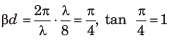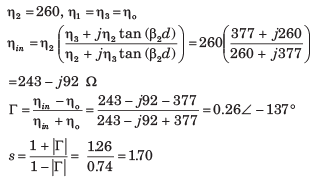Test: Electromagnetic Wave Propagation - Question 19

The E-field of a uniform plane wave propagating in a dielectric medium is given by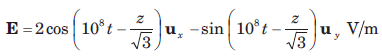The dielectric constant of medium is

Detailed Solution for Test: Electromagnetic Wave Propagation - Question 19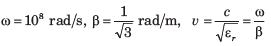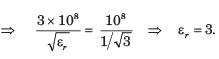Test: Electromagnetic Wave Propagation - Question 20

An electromagnetic wave from an under water source with perpendicular polarization is incident on a water-air interface at angle 20o with normal to surface. For water assume εr = 81, μr = 1. The critical angle θc is

Detailed Solution for Test: Electromagnetic Wave Propagation - Question 20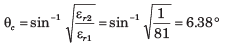## GATE ECE (Electronics) 2023 Mock Test Series

20 docs|261 tests
 Use Code STAYHOME200 and get INR 200 additional OFF Use Coupon Code
Information about Test: Electromagnetic Wave Propagation Page
In this test you can find the Exam questions for Test: Electromagnetic Wave Propagation solved & explained in the simplest way possible. Besides giving Questions and answers for Test: Electromagnetic Wave Propagation, EduRev gives you an ample number of Online tests for practice

## GATE ECE (Electronics) 2023 Mock Test Series

20 docs|261 tests